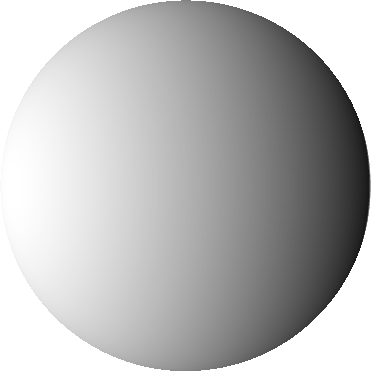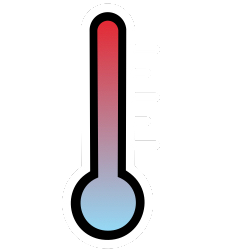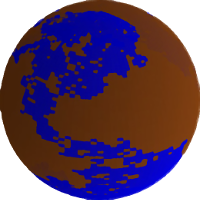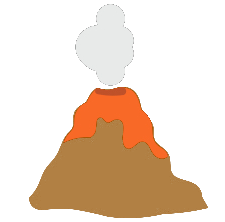New World 32.5℃ 0.10 6.48 0.38
Earth 14.9℃ 0.29 1.0 0.19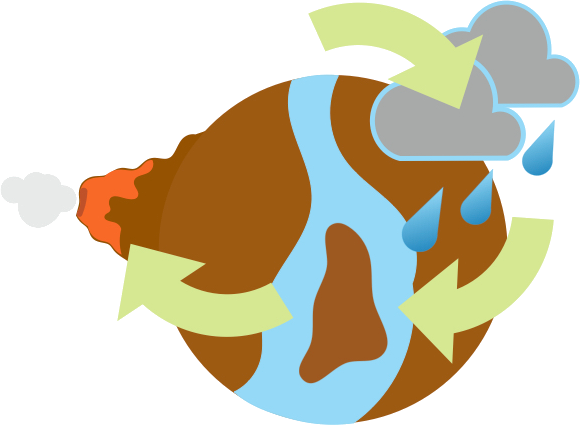# Earth-Like

(Background: ESA / Digitized Sky Survey 2)

Exactly how "Earth-like" is an Earth-like planet?

We have discovered 4455 exoplanets; planets orbiting stars other than the Sun. Many of these are close in size to the Earth, spouting claims that we have discovered "Earth 2.0": a habitable planet whose environment is similar to the present-day Earth. In truth, the average size is all we currently know about most of these new worlds.

But what if we did know much more? An Earth-sized planet with the same rock type, atmospheric composition and water content would certainly be Earth-like, but would even this definitely be a twin to our home world?

EARTH-LIKE is a challenge to explore that question.

Vary the land fraction, volcanism and the position within the habitable zone; all factors that have varied during the Earth's own history. Does the resulting surface temperature suggest a world really like where we live, or a truly alien concoction?

EARTH-LIKE also produces a unique rendition of how your Earth-like planet might appear. A landmap is created based on your chosen fraction of land, volcanism rate and the resultant surface temperature and then a neural network renders this into a realistic landscape. The result is a different world above each time you build a new planet.

If you can find wildly different environments on Earth-like planets only slightly different to our own, just imagine the diversity in the planets we have discovered. Finding out about their environments is going to be an incredible journey.## The SciencePlanet Thermostat

#### The Carbon Cycle

On geological timescales of a few million years, the Earth can regulate its surface temperature by changing the levels of carbon dioxide in the atmosphere. Carbon dioxide is a greenhouse gas that (like a garden greenhouse) can trap the heat our planet receives from the Sun. The more carbon dioxide in the atmosphere, the warmer the planet. The level of carbon dioxide is controlled by the 'carbon cycle' (or 'carbon-silicate cycle'). This is one of the Earth's geochemical cycles, of which the most famous example is the water cycle where water evaporates from the ocean, falls as rain and then returns to the ocean via rivers or ground water. Just like water, carbon makes a cycle and three of the controlling factors just built your Earth-like planet.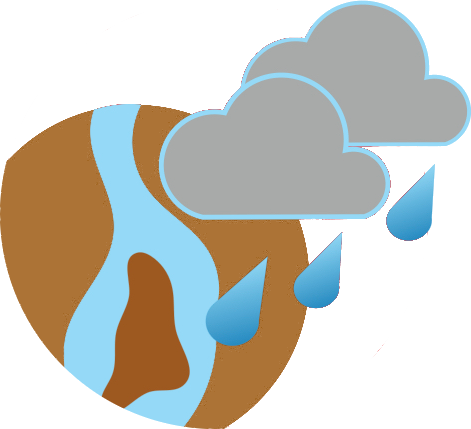Land Fraction

#### Weathering

Carbon dioxide in the atmosphere dissolves in rain water and reacts with continental rocks on exposed land to create hydrogen carbonate. This is known as 'weathering'. In this model, weathering is proportional to the amount of exposed land (land fraction) and the surface temperature, as chemical reactions such as weathering occur faster in warmer conditions. A planet with a larger fraction of exposed land will be able to pull carbon dioxide more rapidly from the air. Experimentation will show you that unless you can replace the carbon dioxide very quickly, a desert world will be fiercely cold. A near-ocean world will slow weathering to a trickle, allowing carbon dioxide to build up in the planet's atmosphere. In reality, a lower level of weathering can occur on the ocean floor, but only if the sea is not too deep.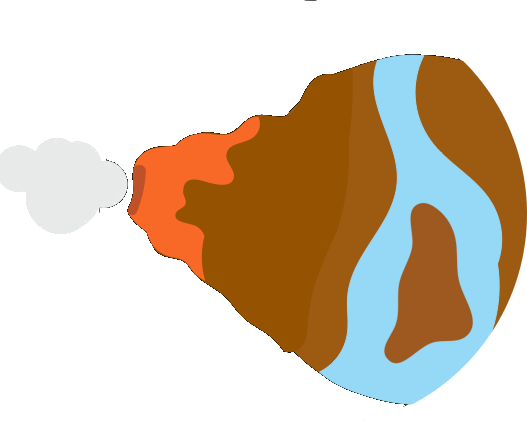Volcanism

#### Outgassing

The hydrogen carbonate dissolves in water and is flushed into the ocean via rivers and ground water. In the ocean, the negatively-charged hydrogen carbonate reacts with positive ions (positively charged elements) to produce salts. These sink and become a solid sediment in a process called 'sedimentation.' Further chemical reactions in the sediment create carbon dioxide and this returns to the atmosphere during volcanic eruptions and other forms of outgassing. The outgassing rate depends on factors such as chemical composition, geological structure and how material moves within the planet. The Earth's outgassing rate has changed over our planet's history. It seems likely that even if we found another planet nearly identical to our own, its outgassing rate might have a very different history. You can see for yourself that this can produce very alien surface conditions.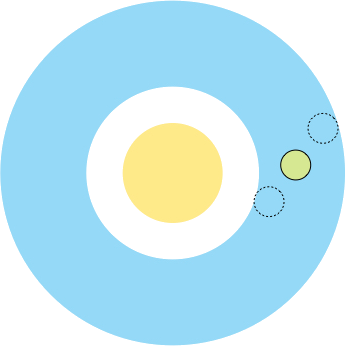Habitable Zone

#### Insolation Level

The surface temperature of the planet depends not just on the contents of the atmosphere, but also on the amount of heat received from the star. In searches for exoplanets that might be habitable, we often talk about the 'habitable zone' or 'goldilocks zone'. This is the region around the star where the level of radiation is suitable to maintain liquid water on the planet's surface. The location of this region depends heavily on planet conditions; a different composition of atmospheric gases, for example, would result in different capabilities to trap heat and make the ideal position for the planet to be closer or further from the star. Since we do not know yet what these exoplanets are like, we usually define the habitable zone as the region where the Earth could use its carbon cycle to adjust the surface temperature to allow liquid water to persist. However, even though the Earth can maintain liquid water within the habitable zone, you can prove that the surface conditions on very similar Earth-like worlds can be very different.

## The Model

Your browser does not support HTML5 Canvas, please update for a better experience.

##### Key idea:

The climate model you just ran moves carbon between three reservoirs on your planet: the atmosphere, the ocean and the solid sediment. This is the carbon cycle described above. Carbon moves between these three reservoirs at rates that depend on your chosen parameters. The weathering rate (that moves carbon from the atmosphere to the ocean) depends on the land fraction and average surface temperature. Meanwhile, volcanism & degassing returns carbon from the sediment to the atmosphere and is affected by your choice for your planet's volcanism. The sedimentation rate moves carbon from the ocean to the sediment, and changes depending on the fraction of carbon stored in the ocean (altered by weathering).

The picture above shows a diagram of this model. The arrow size and colour for weathering and volcanism & degassing change depending on your parameter choices. Fatter arrows represent a larger initial flux of carbon between reservoirs, while blue and red indicates a cooling or warming effect compared to present-day Earth (the sedimentation flow is initially always the same as present-day Earth, but will change in proportion to the abundance of carbon in the ocean increasing or decreasing). Put your planet closer to the inner habitable zone edge or give the planet more land, and the weathering rate will increase to cool the planet. Bump up the volcanism and the planet will start to warm as carbon is pumped into the atmosphere. Eventually, these fluxes will balance and your planet will reach its new average surface temperature.

The average surface temperature is calculated from the amount of carbon in the atmosphere (which forms the greenhouse gas, carbon dioxide) and the radiation from the star (determined your choice of position within the habitable zone). The surface temperature also assumes additional heating from water vapour, which is another greenhouse gas.

##### Shuffling carbon:

Your planet initially begins as a present day Earth, with the carbon reservoirs and surface temperature taken from global estimates. Your choices for your new planet properties changes the weathering and volcanism rates and carbon starts to move between the reservoirs. This alters the amount of carbon in the atmosphere and the surface temperature changes. Eventually, the carbon cycle reaches a new balance point where the amount of carbon entering and leaving each reservoir becomes constant. We call this point equilibrium. The surface temperature at equilibrium is the new global temperature of your Earth-like world.

You can see your planet evolve to its new state on the plot to the right. The ocean and sediment reservoirs contain the greatest quantities of carbon, so they change relatively little compared to the atmosphere.

Depending on how different your planet is from the Earth today, it may take a long or short time to reach equilibrium. If the graph never flattens to a constant value, then equilibrium has not been achieved within the time limit for this model. The surface temperature is then a lower or upper limit (depending on if the graph is rising or falling).

You can download the data of your planet's evolution by pressing the "Download data" button underneath the graph. This is handy if you want to compare models by plotting them yourself. Due to disc space, the data is available for 5 minutes after the page is loaded. Need it again? Just reload the page.

##### Of note:

This model must always have three carbon reservoirs, so it is not actually possible to consider a completely dry planet or an ocean world with no exposed land. In the case where land fraction is 1.0, it can be assumed there is water beneath the surface providing the reservoir. For a near-ocean world (very tiny land fraction), weathering is throttled so the code may never reach equilibrium. The temperature recorded is therefore a lower limit and the planet will actually get hotter. In reality, secondary mechanisms could kick-in on ocean worlds, such as evaporation exposing land once again. You might also be interested in checking out our F.A.Q. for other fun facts about this model.

##### Show me the maths!

If this description was insufficient to quench your curiosity, we can describe the model quantitively. The carbon in each reservoir (R) is found by integrating the following four equations over time:

${dR_{\rm atm} \over dt} = F_{\rm vol} - F_{\rm w}$ ${dR_{\rm oce} \over dt} = F_{\rm w} - F_{\rm sed}$ ${dR_{\rm sed} \over dt} = F_{\rm sed} - F_{\rm vol}$ ${dR_{\rm atm} \over dt} + {dR_{\rm oce} \over dt} + {dR_{\rm sed} \over dt}= 0$ The first three equations describe how carbon changes in each reservoir over time. The atmosphere reservoir ($$R_{\rm atm}$$) gains carbon due to the flux from volcanism & degassing ($$F_{\rm vol}$$) and loses it through the flux due to weathering ($$F_{\rm w}$$). Similarly, the ocean reservoir ($$R_{\rm oce}$$) gains carbon from weathering but loses it through sedimentation ($$F_{\rm sed}$$). Finally, the sediment reservoir gains carbon from sedimentation and loses it through volcanism & degassing ($$F_{\rm vol}$$). The final equation says all of these changes need to add to zero, as no carbon is lost or gained from the planet.

Weathering is the most complex of the fluxes, as it depends on the land fraction, $$\gamma$$, the abundance of carbon in the atmosphere reservoir, $$R_{\rm atm}$$, and the surface temperature, $$T_s$$. The form is the same as in previously published works such as Abbot et al. (2012): $F_{\rm w} = F_{\rm w, \oplus}\left(\frac{\gamma}{\gamma_\oplus}\right)\left(\frac{R_{\rm atm}}{R_{\rm atm,\oplus}}\right)^\beta e^{(T_s - T_{s,\oplus})/k}$ The parameters with a $$\oplus$$ subscript denote a constant equal to that value on present-day Earth (the starting point for the new world). The index $$\beta = 0.165$$ is a rate constant that controls how sensitive the weathering is to the abundance of carbon in the atmosphere. Similarly, $$k = 10$$K ($$-254.15^\circ$$C) and controls how sensitive the weathering is to the surface temperature.

The surface temperature is calculated from the abundance of carbon in the atmosphere via a parameterisation developed by Walker et al. (1981): $T_s = T_{s, \oplus} + 2(T_{\rm eq} - T_{\rm eq, \oplus}) + 4.6\left(\frac{R_{\rm atm}}{R_{\rm atm, \oplus}}\right)^{0.364} - 4.6$ $$T_{\rm eq}$$ is the temperature of the planet at the top of the atmosphere, i.e. the temperature purely based on the radiation from the star without the effect of the greenhouse gases in the atmosphere. For the Earth, our $$T_{\rm eq}$$ is a very chilly $$-18^\circ$$C, but our atmosphere traps heat to give a global surface average of about $$15^\circ$$C. The equilibrium temperature is given by: $T_{\rm eq} = (1 - \alpha)\left(\frac{L_\odot}{16\pi\sigma a^2}\right)^{1/4}$ Here, $$\alpha$$ is the albedo or reflectivity of the planet, which determines how much radiation is reflected back into space. For the Earth $$\alpha = 0.3$$. This value rises as the average surface temperature drops below 273.15K ($$0^\circ$$C) and the planet begins to become covered with ice. Below 250K ($$-23^\circ$$C), the albedo reaches 0.6, a value consistent with an icy world such as the Jovian moon, Europa.

The fluxes for sedimentation and volcanism & outgassing are more simply proportional to the abundance of carbon in the oceans and sediment, multiplied by the rate at which carbon is leaving the atmosphere on present-day Earth (weathering, $$F_{\rm w, \oplus}$$): $F_{\rm sed} = F_{\rm w, \oplus}\frac{R_{\rm oce}}{R_{\rm oce, \oplus}}$ $F_{\rm vol} = \Gamma F_{\rm w, \oplus}\frac{R_{\rm sed}}{R_{\rm sed, \oplus}}$ The rate of volcanism & degassing can be increased or slowed by the factor $$\Gamma$$ that is your chosen volcanism in the model.

A examination of the model performance and how the surface temperature varies with difference choices for the planet conditions can be found in the Earth-like paper, published in the International Journal of Astrobiology (Tasker et al. IJA 2020: preprint here). Our F.A.Q. is also worth a look.

## Landscaping a planetAn imagined surface for your new planet is sketched out when you hit the [Render my planet] button.

Smacking that button creates a landmap, with a random distribution of continents and ocean in a ratio equal to your land fraction choice. The colours of the land reflect both the global surface temperature and level of volcanism. Colder planets have an ice cap that creeps down towards the equator. Land not covered by ice has either green, yellow or grey hues. These are randomly selected, but weighted by the level of volcanism; a more volcanic world will be more likely to select a grey pixel to represent the raised land of an explosive planet. These pixelated values are then converted by a neural network into a realistic looking image of a planet's surface, based on images of the variety of landscape we have on Earth.

While we currently have no way of knowing what the surface of an exoplanet might truly be like, it is fun to imagine the visage that might greet us on an Earth-like world around another star.

## @EarthLikePlanet

Desperate for a new Earth-like planet? We understand. Our twitter bot will provide you with a new planet around the clock! The account tweets a randomised planet a few times a day and responds to tweets with a tailor-made world when the land fraction, volcanism and habitable zone position are specified.

For example, "@EarthLikeWorld I'd like a new planet with a land fraction of 0.3, volcanism 1.0 and habitable zone position of 0.8!"

Why not give it a go?

## The Earth-Like Team#### Kana Ishimaru

Lunar and Planetary Laboratory

University of ArizonaInstitute of Space and Astronatical Science (ISAS)

Japan Aerospace Exploration Agency (JAXA)#### Nicholas Guttenberg

Earth-Life Sciences Institute (ELSI)

Tokyo Insitute of Technology#### Julien Foriel

Dept. of Earth & Planetary Sciences

Harvard University

Earth-Like is a project from the ELSI Origins Network Planetary Diversity Workshop.